# Maharashtra Board 9th Class Maths Part 2 Problem Set 8 Solutions Chapter 8 Trigonometry

Balbharti Maharashtra State Board Class 9 Maths Solutions covers the Problem Set 8 Geometry 9th Class Maths Part 2 Answers Solutions Chapter 8 Trigonometry.

## Problem Set 8 Geometry 9th Std Maths Part 2 Answers Chapter 8 Trigonometry

Question 1.
Choose the correct alternative answer for the following multiple choice questions.

i. Which of the following statements is true?
(A) sin θ = cos (90 – θ)
(B) cos θ = tan (90 – θ)
(C) sin θ = tan (90 – θ)
(D) tan θ = tan (90 – θ)
(A) sin θ = cos (90 – θ)

ii. Which of the following is the value of sin 90°?
(A) $$\frac { \sqrt { 3 } }{ 2 }$$
(B) 0
(C) $$\frac { 1 }{ 2 }$$
(D) 1
(D) 1

iii. 2 tan 45° + cos 45° – sin 45° = ?
(A) 0
(B) 1
(C) 2
(D) 3
2 tan 45° + cos 45° – sin
$$=2(1)+\frac{1}{\sqrt{2}}-\frac{1}{\sqrt{2}}=2$$
(C) 2

iv. $$\frac{\cos 28^{\circ}}{\sin 62^{\circ}}$$ =?
(A) 2
(B) -1
(C) 0
(D) 1
$$\frac{\cos 28^{\circ}}{\sin 62^{\circ}}$$(D) 1

Question 2.
In right angled ∆TSU, TS = 5, ∠S = 90°, SU = 12, then find sin T, cos T, tan T. Similarly find sin U, cos U, tan U.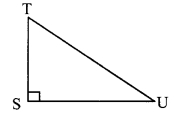Solution:
i. TS = 5, SU = 12 …[Given]
In ∆TSU, ∠S = 90° … [Given]
∴ TU2 = TS2 + SU2 …[Pythagoras theorem]
= 52 + 122 = 25 + 144 = 169
∴ TU = $$\sqrt { 169 }$$ .. .[Taking square root of both sides]
= 13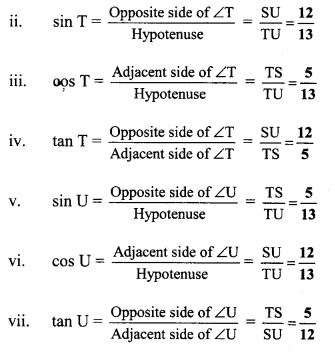Question 3.
In right angled ∆YXZ, ∠X = 90°, XZ = 8 cm, YZ = 17 cm, find sin Y, cos Y, tan Y, sin Z, cos Z, tan Z.Solution:
i. XZ = 8 cm, YZ = 17 cm …[Given]
In ∆YXZ, ∠X = 90° … [Given]
∴ YZ2 = XY2 + XZ2 .. .[Pythagoras theorem]
∴ 172 = XY2 + 82
∴ 289 = XY2 + 64
∴ XY2 = 289 – 64
= 225
∴ x = $$\sqrt { 225 }$$ .. .[Taking square root of both sides]
= 15Question 4.
In right angled ∆LMN, if ∠N = θ, ∠M = 90°, cos θ = $$\frac { 24 }{ 25 }$$, find sin θ and tan θ. Similarly, find (sin2θ) and (cos2θ).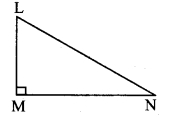Solution:
i. cos θ = $$\frac { 24 }{ 25 }$$
In ∆LMN, ∠M = 90°, ∠N = θ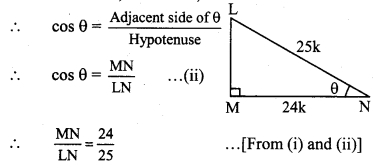Let the common multiple be k.
∴ MN = 24k and LN = 25k
Now, LN2= LM2 + MN2 … [Pythagoras theorem]
∴ (25k)2 = LM2 + (24k)2
∴ 625 k2 = LM2 + 576k2
∴ LM2 = 625k2 – 576k2
∴ LM2 = 49k2
∴ LM = $$\sqrt { 49{ k }^{ 2 } }$$ .. .[Taking square root of both sides]
= 7k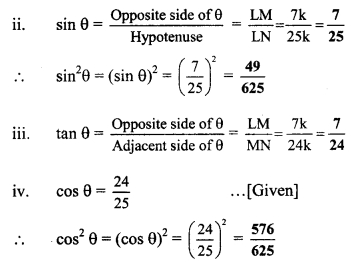Question 5.
Fill in the blanks.
i. sin 20° = cos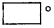ii. tan 30° x tan= 1
iii. cos 40° = sinSolution:
i. sin 20° = cos (90° – 20°) …..[∵ sin θ = cos (90 – θ)]
= cos 70°

ii. tan θ x tan (90 – θ) = 1
Substituting θ = 30°,
tan 30° x tan (90 – 30)° = 1
∴ tan 30° x tan 60° = 1

iii. cos 40° = sin (90° – 40°) …[∵ COS θ = sin (90 – θ)]
= sin 50°

Maharashtra Board Class 9 Maths Solutions Chapter 8 Trigonometry Problem Set 8

Question 1.
Measuring height of a tree using trigonometric ratios. (Textbook pg. no. 101)This experiment can be conducted on a clear sunny day. Look at the figure given above. Height of the tree is QR, height of the stick is BC.
Thrust a stick in the ground as shown in the figure. Measure its height and length of its shadow. Also measure the length of the shadow of the tree. Using these values, how will you determine the height of the tree?
Solution:
Rays of sunlight are parallel.
So, ∆PQR and ∆ABC are equiangular i.e., similar triangles.
Sides of similar triangles are proportional.
∴ $$\frac { QR }{BC }$$ = $$\frac { PR }{ AC }$$
∴ Height of the tree (QR) = $$\frac { BC }{ AC }$$ x PR
Substituting the values of PR, BC and AC in the above equation, we can get length of QR i.e., the height of the tree.

Question 2.
It is convenient to do the above experiment between 11:30 am and 1:30 pm instead of doing it in the morning at 8’O clock. Can you tell why? (Textbook pg. no. 101)
Solution:
At 8’O clock in the morning, the sunlight is not very bright. At the same time, the sun is on the horizon and the shadow would by very long. It would be extremely difficult to measure shadow in that case.
Between 11:30 am and 1:30 pm, the sun is overhead and it would be easier to measure the length of shadow.

Question 3.
Conduct the above discussed activity and find the height of a tall tree in your surrounding. If there is no tree in the premises, then find the height of a pole. (Textbook pg. no. 101)